Specimen Size, Slenderness Ratio and Loading Rate Effect on Dynamic Simulation of HPC | OMICS International
Journal of Civil & Environmental Engineering

# Specimen Size, Slenderness Ratio and Loading Rate Effect on Dynamic Simulation of HPC

Visit for more related articles at Journal of Civil & Environmental Engineering

#### Abstract

The length to diameter ratio (L/D), loading rate, size and specimen geometry are significant factors that affect the compressive strength of high performance concrete (HPC) under dynamic loading conditions. A numerical study is carried out on selected conventional laboratory size prismatic specimen between the slenderness ratio 0.5 to 3.5 to evaluate the variation of stress-strain by performing finite element (FE) solving procedure. The results show that the maximum stress is found in specimen with slenderness ratio one. The stresses gradually decreased with the increase of the value of slenderness ratio (from one and above). The HPC dynamic strength dependency on strain rate and L/D is correlated using multiple regression equation. The accuracy of the predicted regression equation is verified with published experimental data and the result is 3% and 25% deviation under the applied strain rate 100 and 300 s -1 respectively. The regression equation yields significant result within the limited stain rate 150 s -1 . The size effect analyses also satisfy existing published experimental data of concrete.

 Keywords Dynamic load; Finite element; High performance concrete; Slenderness ratio; Stress-strain; Size effect; Loading rate Introduction Many experimental and theoretical investigations have showed that behavior of concrete loaded in tension, compression, shear or torsion is largely influenced by the specimen size and loading rate. Numerous researches were performed to understand the effect of fracture characteristics on material properties of concrete to evaluate the load carrying capacity. The results of these researches were inconsistence due to the large number of variables that affect the results such as specimen size, geometry, length to diameter ratio (L/D) as well as moisture content in the specimens, concrete quality, curing procedure and age [1-3]. The most effective experimental method is to determine the size effect. The term size effect means concrete material strength dependency on specimen size variation. Concrete compressive strengths are mostly evaluated by tests performed on cylindrical specimens with slenderness ratio of two. Nevertheless, when it is necessary to evaluate in situ concrete strength (drilled cores); specimen with slenderness ratio lower than two can be used. Added to this fact, other type of specimens, that is prism or cube has been adopted in many countries, due to the greater amount of experimental work based on them. Over the last 10 years, significant size effect studies on compressive failure of concrete were performed by many researchers in static loading conditions [4-9]. In their study Kim et al. , the numbers of specimens tested with height to diameter ratio L/D=2.0 and L/D ≠ 2 are 222 and 456, respectively. The failure stress of a series of concrete prism is expressed by the following equation :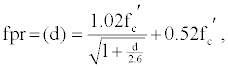(1) where compressive strength of prisms, fpr(d), compressive strength, f ’c, are in MPa and the diameter of cylinder or in case of prism, the smallest side of the cross section of prism, d, is in cm. These studies are based on the static loading conditions and the maximum size of coarse aggregate is 13 mm. It is well known that the load carrying capacity increases substantially at higher strain rates. The way of loading rate and the size effect phenomena interact with each other, however, is not well understood. Elfahal et al. [10-12] have investigated the size effect phenomenon numerically and experimentally on both normal and high strength concrete cylinders subjected to static and dynamic compression within the limited strain rates of 0.111 s-1 and below. Few or little information is available on effect of L/D to the compressive strength of concrete subjected to high rate of loading. There is still a lack of knowledge on the HPC response in dynamic loading conditions like blast or impact loading [11,12]. Analysis on behavior of concrete under dynamic loading conditions are quite complicated and the parameters that can affect the results include the inertia effects, specimen geometry, uniformity of stress and strain along the specimen length and wave propagation effects. The effect of L/D on the dynamic behavior becomes particularly important at very high rates of loading. In this study, dynamic strength dependency on different L/D specimens and loading rate are presented. The regression model between dynamic strength with different L/D specimens and loading rate developed can be used in predicting the dynamic behavior of concrete accurately. Also, this paper contributes by discussing the stress-strain behavior of HPC under the dynamic load regarding to the influence of size. Different modes of failure at the extreme loading such as greater than 1300 GPa/s are also described. Material and Concrete Mixes The static properties of HPC incorporating silica fume used for simulation in this research was determined by Ilham . The ingredients, details of mixing proportions and properties are shown in Table 1. Finite Element Modelling A non-associated plastic flow rule along with combination of isotropic properties of elasticity is used on the whole structure of concrete specimen. The Drucker-Prager linear function is used to define the plastic flow potential. The strength hardening used to trace the evaluation of the yield surface is the effective plastic strains in compression and the start of compressive plastic strains in these numerical analyses are considered to be zero. The physical model is formed with two parts namely bearing plate and concrete prism. Details descriptions of the modeling of concrete are illustrated in the subsections 3.1-3.4 and the block diagram of the model is shown in Figure 1. Geometry of the specimens Numerical studies are performed on selected conventional laboratory size prismatic specimens of L/D 0.5 to 3.5 conforming to the experimental specimens used by Cotsovos and Pavlovic  and cylindrical specimens of L/D are equal to 2 following investigators del Viso et al. , Krauthammer et al.  and Elfahal et al. . The diameter of a cylinder is equivalent to the least dimension of the cross section of a prism. In this study, square cross section is opted, so that D is equivalent to either depth or width of the square. The detailed dimensions of the prismatic and cylindrical specimens are summarized in Table 2. Rigid body and contact A general contact algorithm is selected between the concrete prism and steel bearing plate (rigid body). The bearing platen bottom surface is defined as the master surface due to dynamic load applied on the top surface; whereas the concrete surface in contact is define as the slave surface. Tie constraint is used to interlock the interface of two contact surfaces for transmission of shear and normal forces across the interface properly. A mechanical interaction model excluding friction loss is defined to model the contact between the bearing plate and concrete surface. A hard contact is used in ABAQUS to formulate the tangential and normal forces in the present model. A central difference rule is used to integrate the equation of motion explicitly through time. The schematic diagram of the simulation setup is shown in Figure 2. Load and boundary condition The bottom face of concrete prism is assumed to be fixed and high magnitude time dependent pressure load from 500 to 2200 GPa/s are imposed on the top face of the bearing plate. In this model, no separation is permitted between the bearing plate-concrete prism contact interfaces to avoid failure occurrence near the top face of concrete specimen. The load is allowed to all three degrees of freedom to avoid the wave deflection problems on the boundaries of the prism. In order to vary the rate of loading, the load increase is kept constant and the time step is varied. Numerical modeling The simulations are conducted using the general purpose finite element code ABAQUS/Explicit version 6.7.2 . The analysis is performed employing a 3D material model to account for the triaxial behavior of concrete. Both the concrete prism and bearing plate are modeled using Lagrangian brick element. Meshes of both concrete prism and bearing plate are selected as structural hex shape, geometric linear and element type C3D8R (An 8-node linear brick, reduced integration, hourglass control). Finer meshes are preferred during the simulation procedure though Cotsovos and Pavlovic  in part I and II adopted coarse meshes in his material model. Usually, dense FE meshes are preferred in order to model the concrete specimen with several investigations adopting FE analysis as small as 2-3 mm [16,17]. Hence, finer meshes are adopted in the present study. In order to obtain more accurate results the concrete specimen is meshed into 3 mm size. Finer meshes are examined in several trail simulations to obtain the most accurate results. Numerical Results and Discussions The results are presented on the basis of the affecting factors, i.e., L/D ratio, loading rate and size. The discussion will be on, firstly, the effect of the specimen length to diameter ratio at different loading rate (the loading rate that produce the highest load bearing capacity) to the stress-strain diagram, next, the effect of L/D at different loading rate, then, the correlation between dynamic strength, L/D and strain rate and finally, the failure patterns at the extreme loading, i.e. greater than 1300 GPa/s. Size effect is discussed through simulations of different sizes of cylindrical specimens at L/D equal to 2. Effect of L/D ratio at different loading rate on stress-strain diagram The stress and strain relationship simulation of the HPC prismatic specimens is presented graphically in Figure 3. The curve for each L/D presented in Figure 3 are taken as the highest load carrying capacity it can take from simulation of each specimen with different L/D at different loading rate between 500-2200 GPa/s The results clearly show that axial stresses increase when the L/D is raised from 0.50 to 1.00 but further increase of the L/D (from 1.50 to 3.50) had resulted in the reduction of the ultimate strength capacity. In addition, Figure 3 also reveals that strains of HPC increase when L/D is raised from 0.50 to 1.5 but the reverse occurred when L/D is raised from 1.50 to 3.50. The failure of the specimens with small slenderness ratio of L/D 0.50 and L/D 0.75 occur at strain value 0.0003 and 0.0007 at maximum stress 129 and 260 MPa respectively. The important factor not shown in Figure 3 is that the extreme load carrying capacity for these two specimens (L/D 0.50 and L/D 0.75) are found to be 2200 GPa/s and 2000 GPa/s accordingly. The specimens with L/D 0.50 and L/D 0.75 are applicable as bearing seats of girder in bridge, thus explained the high load bearing capacity they can held. On the other hand, the load carrying capacities for the other specimens (L/D 1.0-L/D 3.5) are found to be at loading rate 1300 GPa/s. The effect of different loading rate (500, 900 and 1300 GPa/s) are clearly found in all sets of results of different L/D. Figure 4 reveals that with the increase of loading rate, stresses increase i.e., the strength at the loading rate 900 GPa/s is nearly 85% higher than at 500 GPa/s in the each sample of the specimen. Furthermore, at 1300 GPa/s applied loading rate, the stresses are on average 138% and 28.5% higher than the stresses at applied loading rate of 500 GPa/s and 900 GPa/s respectively. The only exception is found in case of specimen L/D 0.50, which shows lower strength but it carries the maximum load (2200 GPa/s). The energies applied are high for short specimen (50 mm compared to 100 x 100 mm cross-section) showing that short specimens are better in withstanding high strain rate of loading. The analogous data demonstrates that the maximum stresses are at the slenderness ratio equal to one under the application of different loading. The stresses decrease with increasing value of slenderness ratio (i.e. one and above). Whereas, in the theoretical static loading conditions, according to eq. (1), stresses decrease with the increasing value of slenderness. The divergent observed also can be attributed to difference in the maximum coarse aggregate size used in the concrete mix and the specimen size. In the present work, simulations are carried out based on the properties of concrete with maximum size of coarse aggregate 19 mm. On the contrary, the maximum size of coarse aggregate used is limited to 13 mm in the static analyses. Hence, the influence of maximum coarse aggregate size is reflected in numerical results of the smaller size of specimen L/D 0.50 and L/D 0.75 as shown in Figures 3 and 4. If the results of these two specimens are disregarded, the results of other specimens follow the existing static law as described in eq. (1) earlier only qualitatively due to loading rate is not considered separately. In this research, the effect of L/D and loading rate are considered separately by using a statistical package to formulate a more realistic correlation. Details of this correlation are illustrated as comparative study with the existing published experimental data in Table 3. Correlation between dynamic strength, L/D and strain rate The obtained dynamic strength with L/D from 1 to 3.5 and strain rate range from 10 to 36 s-1 are tabulated in Table 3. The relationships between dynamic strength as a dependent variable and two independent variables of slenderness ratio and strain rates is as expressed by the mathematical regression equation. The values of regression parameters are predicted by using statistical package of “Finite Mathematics & Applied Calculus Resources Page”. The mathematical regression equation is as follows: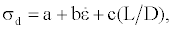(2) where σd is the dynamic strength, a, b and c are regression parameters,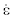and (L/D) are the strain rate and length to diameter ratio respectively. The values of regression parameters are found to be a=-97.00585, b=0.97975 and c=6.60524. Hence, the dynamic strength of concrete can be obtained from the analogous data is expressed by the following equation: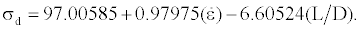(3) Developed regression model eq. (3) reveals the dynamic strength dependency on loading rate at different length to diameter ratio. The results from the predicted regression eq. (3) are compared with experimental results conducted by Riisgaard et al.  with the static strength of concrete 100 MPa in order to verify the accuracy. The experimental test is conducted under the applied strain rate from 100 to 300 s-1 using Split Hopkinson Pressure Bar with the value of L/D is equal to 1 for each specimen. The predicted regression model shows that the deviations between the predicted results and the experimental results are 3%, 24%, 34%, 59% with respect to strain rates 102, 145, 197 and 300 s-1 accordingly (Figure 5). This comparative study demonstrates that the predicted model generates the accurate results with reasonable difference to be 25% under the application of strain rate 150 s-1. For strain rate higher than 150 s-1, the percentage of error is not acceptable. Analysis of failure pattern Several modes of failure are detected during the simulations. The deformable contour shapes prior to failure are shown in Figure 6. The short specimen, that is, L/D 0.50 and L/D 0.75 are found to exhibit cone shaped shear failure as shown in Figure 6A and B. The prism fails at the side forming two cones at the top and bottom in the cone shaped shear failure modes. Compressive belly failures are found in specimens L/D 1.00, L/D 1.50 and L/D 2.00 with the inflation of the prism centre, forming a belly shape as shown in Figure 6C-E. In practical, this type of failure happens combined with the shell-bursting. Buckling failure is distinguished of specimen L/D 3.5 with the inflation of the prism from the top and bulging of material as shown in Figure 6F. This type of failure is observed due to the greater value of slenderness ratio (L/ D=3.5). Specimen size effect Numerical studies are carried out on selected cylindrical specimens with the slenderness ratios of two and the obtained results are summarized in the Table 4. The present numerical results are compared with the existing results with static strength 89 MPa as shown in Table 5. Comparative study demonstrates that differences of 9%, 31%, 39% for the specimens φ75 × 150, φ150 × 300, φ300 × 600 between the present study and the simulated results using Drucker-Prager model performed by Krauthammer et al. (2003). The variation of result occurs due to the loading rate and different method of loading and not satisfying eq. (3) for specimen Cy300. Table 5 shows that predicted simulation results also agree with the dynamic test results rather than the Brittle fracture model. Conclusion Dynamic strength dependency on length to diameter ratio size and loading rate effect of prismatic specimen with slenderness ratios between 0.5 to 3.5 and cylindrical specimen with slenderness ratio of 2.0 of each specimen is studied. From the numerical results the following conclusion can be pointed out; (i) the maximum stresses are found in specimen with slenderness ratio one. (ii) The stresses gradually decreased with the increase of the value of slenderness ratio (from one and above), (iii) three different modes of failure are found: cone shape shear failure; compressive belly failure; and top buckling failure, (iv) The multiple model regression equation shows good agreements with the existing experimental results showing 25% deviation at strain rate 150 s-1, (v) The comparative study with L/D equal to 2 for each cylindrical specimen satisfy the simulation results performed by the investigator Krauthammer et al. 2003. Therefore, it can be concluded that the proposed eq. (3) can described well the dependency of dynamic strength with the rate of loading, size and L/D of specimen. Acknowledgements This research is sponsored by the Ministry of Higher Learning Malaysia under the grant UKM-KK-02-FRGS0014-2006. References Bischoff PH, Perry SH (1991) Compressive behaviour of concrete at high strain rates.J Eng Mech 24: 425-450. Cotsovos DM, Pavlovic MN (2008) Numerical investigation of concrete subjected to compressive impact loading. Part 1: A fundamental explanation for the apparent strength gain at high loading rates. Computers & Structures 86: 145-163. Ross CA, Jerome DM, Tedesco JW, Hughes ML (1996) Moisture and strain rate effects on concrete strength. ACI Materials Journal 93: 293-300. Kim JK, Yi ST, Park CK, Eo SH (1999) Size effect on compressive strength of plain and spirally reinforced concrete cylinders. ACI Structural Journal 96: 88-94. Kim JK, Yi ST, Yang EI (2000) Size effect on flexural compressive strength of concrete specimens. ACI Struct J 97: 291-296. Kim JK, Yi ST and Kim JHJ (2001) Effect of specimen sizes on flexural compressive strength of concrete. ACI Struct J 98: 416-424. Kim JH, Yi ST and Kim JK (2004) Size effect of concrete members applied with flexural compressive stresses. Int J Fracture 126: 79-102. Yi ST, Kim JHJ, Kim JK (2002) Effect of specimen sizes on ACI rectangular stress block for concrete flexural members. ACI Struct J 99: 701-708. Yi ST, Yang E, Choi J (2006) Effect of specimen sizes, specimen shapes, and placement directions on compressive strength of concrete. Nuclear Engineering and Design 236: 115-127. Elfahal MM, Krauthammer T (2005) Dynamic size effect in normal and high strength concrete cylinders. ACI Materials Journal 102: 77-85. Elfahal MM, Krauthammer T, Ohno T, Beppu M, Mindess S (2005) Size effect for normal strength concrete cylinders subjected to axial impact. International Journal of Impact Engineering 31: 461-481. Kruathammer T, Elfahal MM, Lim J, Ohno T, Beppu M et al. (2003) Size effect for high-strength concrete cylinders subjected to axial impact.International Journal of Impact Engineering 28: 1001-1016. Ilham A (2003) Mix design method and properties of high performance concrete for compressive strength between 50 and 100 MPa. Universiti Kebangsaan Malaysia, Malaysia. del Viso JR, Carmona JR, Ruiz G (2008) Shape and size effects on the compressive strength of high-strength concrete. Cement and Concrete Research 38: 386-395. Hibbitt, Karlsson, Srensen (2007) ABAQUS/explicit user manual, USA. Park SW, Xia Q, Zhou M (2001) Dynamic behavior of concrete at high strain-rates and pressures: II. Experimental characterization. Int J Impact engng 25: 887-910. Li QM, Meng H (2003) About the dynamic strength enhancement of concrete-like materials in a split Hopkinson pressure bar test. Int J Solids Struct 40: 343-360. Riisgaard B, Ngo N, Mendis P, Georgakis CT, Stang H (2007) Dynamic increase factors for high performance concrete in compression using Split Hopkinson Pressure Bar. International conference of Fracture Mechanics of Concrete Structures, Technical University of Denmark, Denmark.

#### Tables and Figures at a glanceTable 1 Table 2 Table 3 Table 4 Table 5

#### Figures at a glance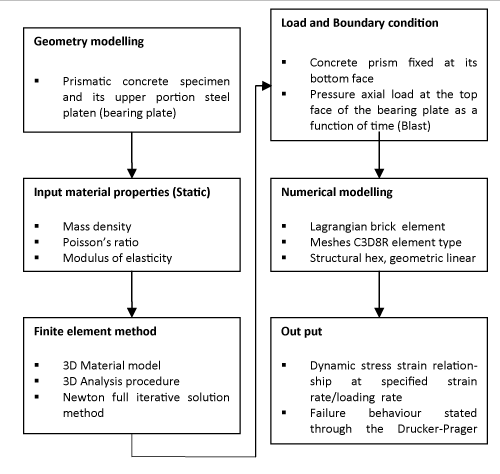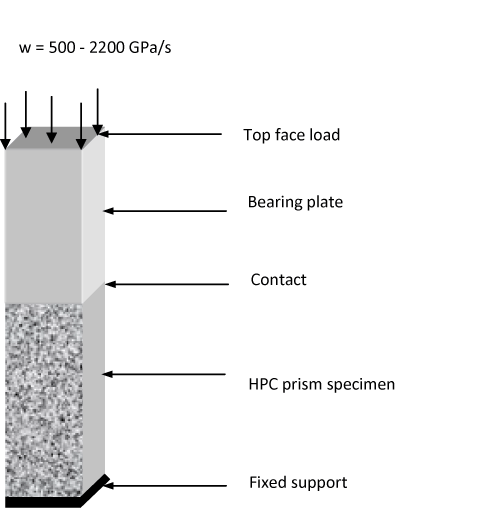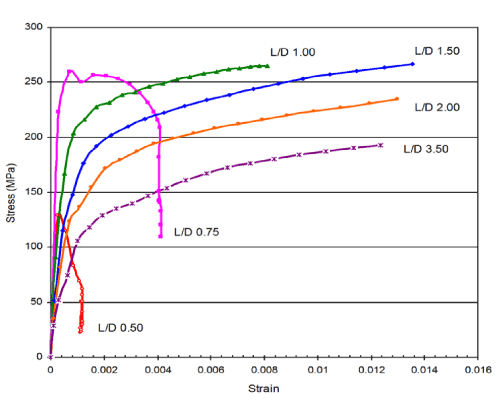Figure 1 Figure 2 Figure 3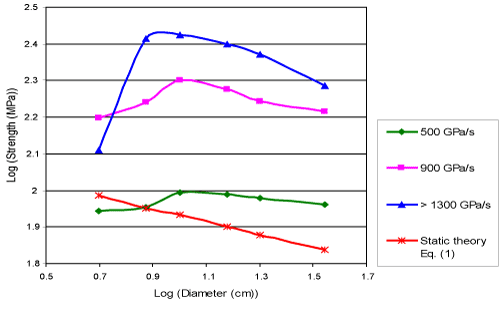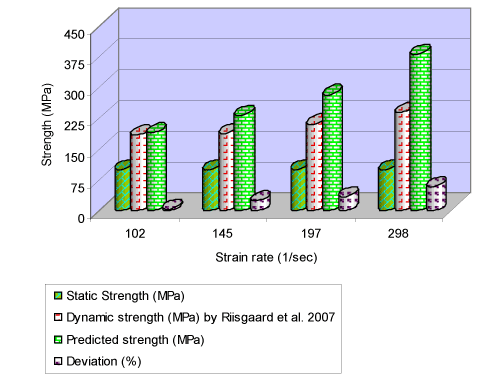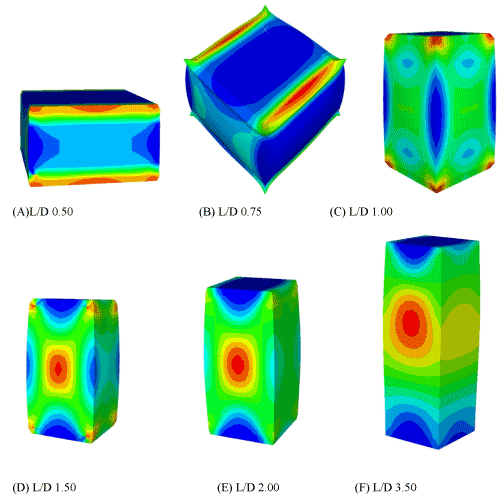Figure 4 Figure 5 Figure 6
Select your language of interest to view the total content in your interested language

### Article Usage

• Total views: 12516
• [From(publication date):
December-2013 - Jan 19, 2020]
• Breakdown by view type
• HTML page views : 8691Can't read the image? click here to refresh